# 【pytest官方文档】解读fixtures - 11. fixture的执行顺序，3要素详解（长文预警）

• scope，就是fixture函数的作用范围，比如scope='class'
• dependencies，可能会存在fixture请求了别的fixture，所以产生了依赖关系，也要考虑进去。
• autouse，如果autouse=True，那么在作用范围内，这个fixture是最先调用的。

### 一、使用范围更大的fixture函数优先执行

import pytest

@pytest.fixture(scope="session")
def order():
return []

@pytest.fixture
def func(order):
order.append("function")

@pytest.fixture(scope="class")
def cls(order):
order.append("class")

@pytest.fixture(scope="module")
def mod(order):
order.append("module")

@pytest.fixture(scope="package")
def pack(order):
order.append("package")

@pytest.fixture(scope="session")
def sess(order):
order.append("session")

class TestClass:
def test_order(self, func, cls, mod, pack, sess, order):
assert order == ["session", "package", "module", "class", "function"]


test_module1.py .                                                        [100%]

============================== 1 passed in 0.01s ==============================
Process finished with exit code 0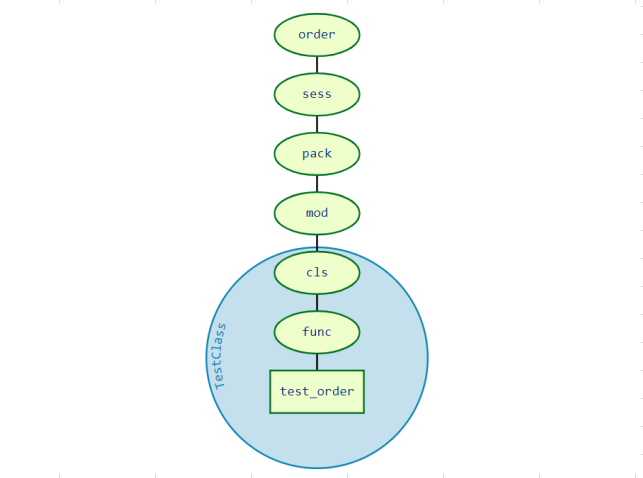### 二、相同顺序的fixture基于依赖项执行

#### 1.请求依赖呈线性情况下

import pytest

@pytest.fixture
def order():
return []

@pytest.fixture
def a(order):
order.append("a")

@pytest.fixture
def b(a, order):
order.append("b")

@pytest.fixture
def c(a, b, order):
order.append("c")

@pytest.fixture
def d(c, b, order):
order.append("d")

@pytest.fixture
def e(d, b, order):
order.append("e")

@pytest.fixture
def f(e, order):
order.append("f")

@pytest.fixture
def g(f, c, order):
order.append("g")

def test_order(g, order):
assert order == ["a", "b", "c", "d", "e", "f", "g"]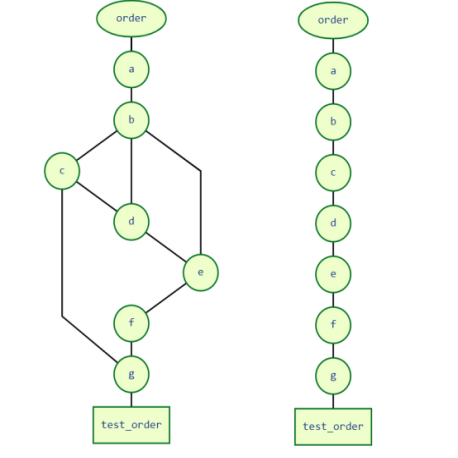#### 2.请求依赖不呈线性的情况，会影响操作执行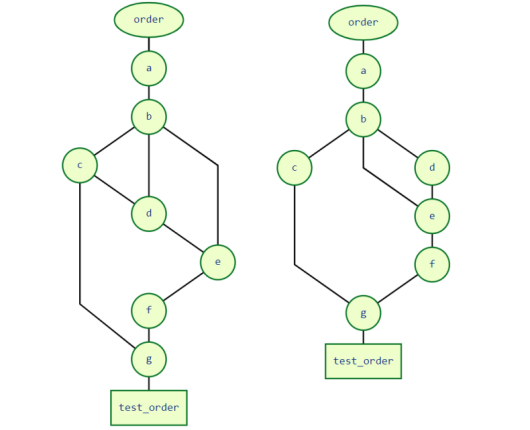• c此时只被一个g请求。
• g既请求了c，还请求了f。

test_module1.py

F
demo\test_module1.py:51 (test_order)
['a', 'b', 'd...'f', 'c', ...] != ['a', 'b', 'c...'e', 'f', ...]

Expected :['a', 'b', 'c...'e', 'f', ...]
Actual   :['a', 'b', 'd...'f', 'c', ...]


### 三、Autouse的fixtures，会优先执行

#### 1. autouse的妙用

import pytest

@pytest.fixture
def order():
print("\n运行order")
return []

@pytest.fixture
def a(order):
print("运行a")
order.append("a")

@pytest.fixture
def b(a, order):
print("运行b")
order.append("b")

@pytest.fixture(autouse=True)
def c(a, b, order):
print("运行c")
order.append("c")

@pytest.fixture
def d(b, order):
print("运行d")
order.append("d")

@pytest.fixture
def e(d, b, order):
print("运行e")
order.append("e")

@pytest.fixture
def f(e, order):
print("运行f")
order.append("f")

@pytest.fixture
def g(f, c, order):
print("运行g")
order.append("g")

def test_order(g, order):
assert order == ["a", "b", "c", "d", "e", "f", "g"]


test_module1.py

.                                                        [100%]

============================== 1 passed in 0.01s ==============================
Process finished with exit code 0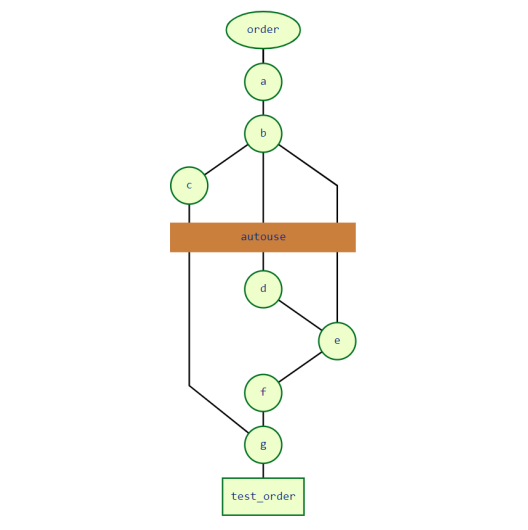#### 2. autouse的慎用

import pytest

@pytest.fixture(scope="class")
def order():
return []

@pytest.fixture(scope="class", autouse=True)
def c1(order):
order.append("c1")

@pytest.fixture(scope="class")
def c2(order):
order.append("c2")

@pytest.fixture(scope="class")
def c3(order, c1):
order.append("c3")

class TestClassWithC1Request:
def test_order(self, order, c1, c3):
assert order == ["c1", "c3"]

class TestClassWithoutC1Request:
def test_order(self, order, c2):
assert order == ["c1", "c2"]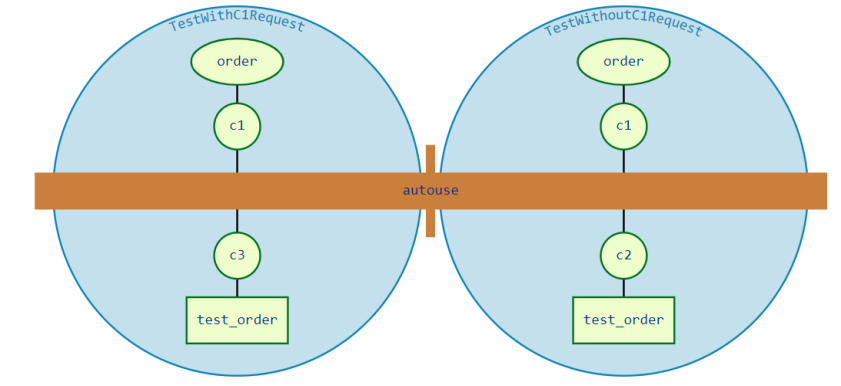import pytest

@pytest.fixture
def order():
return []

@pytest.fixture
def c1(order):
order.append("c1")

@pytest.fixture
def c2(order):
order.append("c2")

class TestClassWithAutouse:
@pytest.fixture(autouse=True)
def c3(self, order, c2):
order.append("c3")

def test_req(self, order, c1):
assert order == ["c2", "c3", "c1"]

def test_no_req(self, order):
assert order == ["c2", "c3"]

class TestClassWithoutAutouse:
def test_req(self, order, c1):
assert order == ["c1"]

def test_no_req(self, order):
assert order == []

if __name__ == '__main__':
pytest.main(['-s', 'test_module2.py'])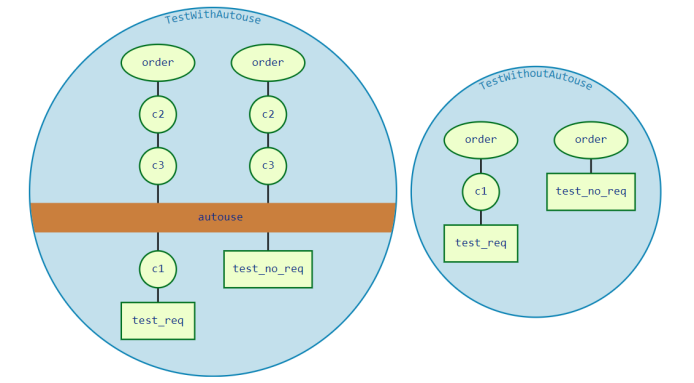• test_reqtest_no_req是2个测试方法。
• c3是autouse，并且请求了c2，所以c2也成了autouse。虽然2个测试并没去请求c2c3，但是都执行了，而且在c1之前执行。

autouse了，所以类TestClassWithoutAutouse中的test_no_req可以运行成功，因为order=[]

posted @ 2021-04-26 00:03  把苹果咬哭的测试笔记  阅读(495)  评论(0编辑  收藏  举报